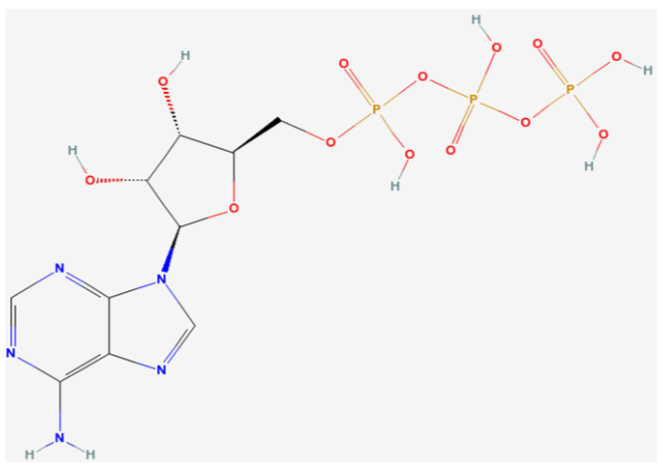# 4.3: Types of Cell Uptake and Transport

•• Contributed by Brian Lindshield
• Associate Prof (Department of Food, Nutrition, Dietetics and Health) at Kansas State University

There are a number of different forms of uptake/transport utilized by your body. These can be classified as passive or active. The difference between the two is whether energy is required and whether (from a solute perspective) they move with or against a concentration gradient. Passive transport does not require energy to move with a concentration gradient. Active transport requires energy to move against the concentration gradient.

##### ADAPT $$\PageIndex{1}$$

The energy for active uptake/transport is provided by adenosine triphosphate (ATP), which is the energy currency in the body. The structures of adenosine and phosphate are shown below.Figure $$\PageIndex{1}$$: Structures of adenosine (left) and phosphate (right)

Tri- means three, thus ATP is adenosine with three phosphate groups bonded to it, as shown below.Figure $$\PageIndex{2}$$: Structure of adenosine triphosphate (ATP)

Phosphorylation is the addition of a phosphate group. Dephosphorylation is removal of a phosphate group. Overall phosphorylation is a process that requires energy. The net effect of dephosphorylation is the release of energy. Thus, energy is required to add phosphates to ATP, energy is released through removing phosphates from ATP.

##### ADAPT $$\PageIndex{2}$$

The concentration gradient is a way to describe the difference between the concentration of the solute outside of a cell versus the concentration inside of a cell. A solute is dissolved in a solvent in a solution; the more solute the higher the concentration. Moving with the gradient is typically moving of solute from a region of higher concentration to an area of lower concentration (in order to reach equal solute concentrations on both sides of the membrane). The exception is osmosis, which moves solvent instead of solute to have the same effect of equalizing concentrations on both sides of the membrane. Moving against the gradient is moving solute from an area of lower concentration to an area of higher concentration.

## Passive Uptake/Transport

There are three forms of passive uptake/transport:

1. Simple Diffusion
2. Osmosis
3. Facilitated Diffusion

### Simple Diffusion

Simple diffusion is the movement of solutes from an area of higher concentration (with the concentration gradient) to an area of lower concentration without the help of a protein, as shown below.Figure $$\PageIndex{3}$$: Simple diffusion

### Osmosis

Osmosis is similar to simple diffusion, but water moves instead of solutes. In osmosis water molecules move from an area of lower solute concentration to an area of higher solute concentration of solute as shown below. The effect of this movement is to dilute the area of higher solute concentration to equalize the solute concentrations on both sides of the membrane.Figure $$\PageIndex{4}$$: Osmosis

The following videos do a nice job of illustrating osmosis.

Another example illustrating osmosis is the red blood cells in different solutions shown below.Figure $$\PageIndex{5}$$: Effect of salt solution concentration on red blood cells1

We will consider the simple example of salt as the solute. If the solution is hypertonic, there is a greater concentration of salt outside (extracellular) the red blood cells than within them (intracellular). Water will then move out of the red blood cells to the area of higher salt concentration, resulting in the shriveled red blood cells depicted. Isotonic means that there is no difference between concentrations. There is an equal exchange of water between intracellular and extracellular fluids. Thus, the cells are normal, functioning red blood cells. A hypotonic solution contains a lower extracellular concentration of salt than the red blood cell intracellular fluid. As a result, water enters the red blood cells, possibly causing them to burst.

### Facilitated Diffusion

The last form of passive absorption is similar to simple diffusion in that it follows the concentration gradient (higher concentration to lower concentration). However, it requires a protein to transport (channel or carrier protein) the solute across the membrane. The following figure and video do a nice job of illustrating facilitated diffusion.Figure $$\PageIndex{6}$$: Facilitated diffusion examples2

## Active Uptake/Transport

There are two forms of active uptake/transport:

1. Active Carrier Transport
2. Endocytosis

### Active Carrier Transport

Active carrier transport is similar to facilitated diffusion in that it utilizes a protein (carrier or transport). However, energy is also used to move compounds against their concentration gradient. The following figure and video do a nice job of illustrating active carrier transport.Figure $$\PageIndex{7}$$: Sodium-potassium ATPase (aka sodium-potassium pump) an example of active carrier transport3

### Endocytosis

Endocytosis is the engulfing of particles, or fluids, to be taken up into the cell in vesicles formed from the cell membrane. If a particle is endocytosed, this process is referred to as phagocytosis. If a fluid is endocytosed, this process is referred to as pinocytosis as shown below.Figure $$\PageIndex{8}$$: Different types of endocytosis4

The following video does a really nice job of showing how endocytosis occurs.

## References

1. en.Wikipedia.org/wiki/File:Os...ls_diagram.svg
2. en.Wikipedia.org/wiki/Facili...embrane-en.svg
3. en.Wikipedia.org/wiki/File:S...um_pump-en.svg
4. commons.wikimedia.org/wiki/Fi...osis_types.svg